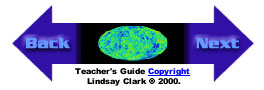Materials: String cut into several different lengths, a few inches, a foot, three feet etc. Protractors A globe, or several if possible A horse's Saddle if possible or try making a hyperbolic sheet from paper or cloth as decribed here. Directions: Have students form small groups. Give each group one of the lengths of string, spreading the different lengths around the room. Have a different length of string for each group. Have the students first make a triangle on a flat piece of paper using the string as the perimeter. Have them measure the angles of the triangle. They should discover that their angles add to 180 degrees. Have them make new triangles on the paper until they are convinced that not matter what shape triangle they form the total angle summation will equal 180 degrees. Now have them make a triangle on the globe using the string as the perimeter. Have them stretch the string as tightly as they can on the surface of the globe. They may find that the sides of their triangle are not straight lines. This is because on the surface of a sphere the shortest distance along the surface is not a straight line. It is actually a portion of what is called a Great Circle. A Great Circle is a circle on the surface of a sphere which has the center of the sphere as its center. For example all of the lines of longitude are Great Circles, but the only line of latitude which is a Great Circle is the Equator. In general terms the shortest distance along any surface is called a geodesic. Have them measure the angles of their triangle and record the sum of the angles. If you have a saddle or hyperbolic sheet, try the same procedure. The geodesic for a hyperbolic surface is a hyperbola. This may not work as well as for the globe because the curvature is not smooth and may have imperfections. See 12. Have the students tabulate their results on a chalkboard in the front of the room for each of the surfaces. See if they can find trends based upon the length of the string and the sum of the angles. If a saddle or hyperbolic sheet are unavailable, have students complete the exercise on the globe and flat piece of paper. Explain that a hyperbolic surface has the opposite result from a sphere. The angles of a triangle on a hyperbolic surface add up to less than 180 degrees.Back | Next